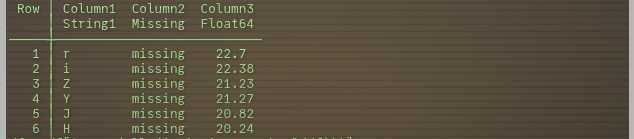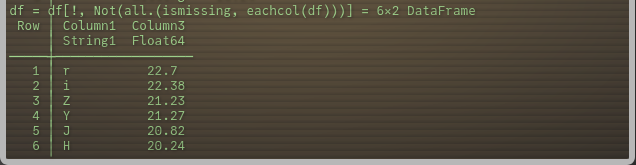# Remove column having missing values and assign default names again

I have a dataframe having default(not given) column names`Column1 Column2 Column3` .and after removing Column containing `missing` values using `df=df[!, Not(all.(ismissing, eachcol(df)))]` it looks likeI want to assign them default column names again so that column names becomes`Column1 Column2` .
I don’t want to rename them manually using `rename!(df, [:Column1, :Column2]`.

I am not sure what you mean by “I don’t want to rename them manually”. Assuming you mean that you don’t want to hard code the names, you could do

``````julia> df = DataFrame(rand(4,3), :auto)
4×3 DataFrame
Row │ x1        x2          x3
│ Float64   Float64     Float64
─────┼─────────────────────────────────
1 │ 0.522163  0.668836    0.808715
2 │ 0.974633  0.729561    0.649561
3 │ 0.236896  0.00306799  0.0709158
4 │ 0.318055  0.758109    0.641894

julia> df2 = select(df, Not(:x2))
4×2 DataFrame
Row │ x1        x3
│ Float64   Float64
─────┼─────────────────────
1 │ 0.522163  0.808715
2 │ 0.974633  0.649561
3 │ 0.236896  0.0709158
4 │ 0.318055  0.641894

rename!(df2, names(df2) .=> names(df)[1 : ncol(df2)])
4×2 DataFrame
Row │ x1        x2
│ Float64   Float64
─────┼─────────────────────
1 │ 0.522163  0.808715
2 │ 0.974633  0.649561
3 │ 0.236896  0.0709158
4 │ 0.318055  0.641894
``````
1 Like

I want to remove any column containing only `missing` values.

A somewhat wordy solution:

``````julia> select(df, [n for n in  names(df) if !all(ismissing, df[!, n])])
2×2 DataFrame
Row │ a      b
│ Int64  Int64
─────┼──────────────
1 │     1      3
2 │     2      4
``````

I can’t think of a less wordy way of doing it. Probably something DataFrames.jl could add eventually tbh.

There is a way to do this with `names`

``````julia> select(df, names(df, (all.(ismissing, eachcol(df))) .== false))
``````
1 Like
``````df[:,Not(names(df,Missing))]
``````
``````DF=df[:,Not(names(df,Missing))]

L=length(names(df,Missing))
N=ncol(df)-L
rename(DF,names(df)[1:N])
``````
1 Like

Is there a reason (or more) for not defining the length of the inverted indexes?

`````` m=names(df,Missing)
# rename(df[:,Not(m)],names(df)[1:ncol(df)-length(m)])
rename(df[:,Not(m)],names(df)[1:length(Not(m))])
ERROR: MethodError: no method matching length(::InvertedIndex{Vector{String}})
``````

@rocco_sprmnt21 Thanks for your answer, but i was able to remove `missing` column by using regex and proper delimiter .

1 Like

Bravo. Prevenire è meglio che curare!

1 Like